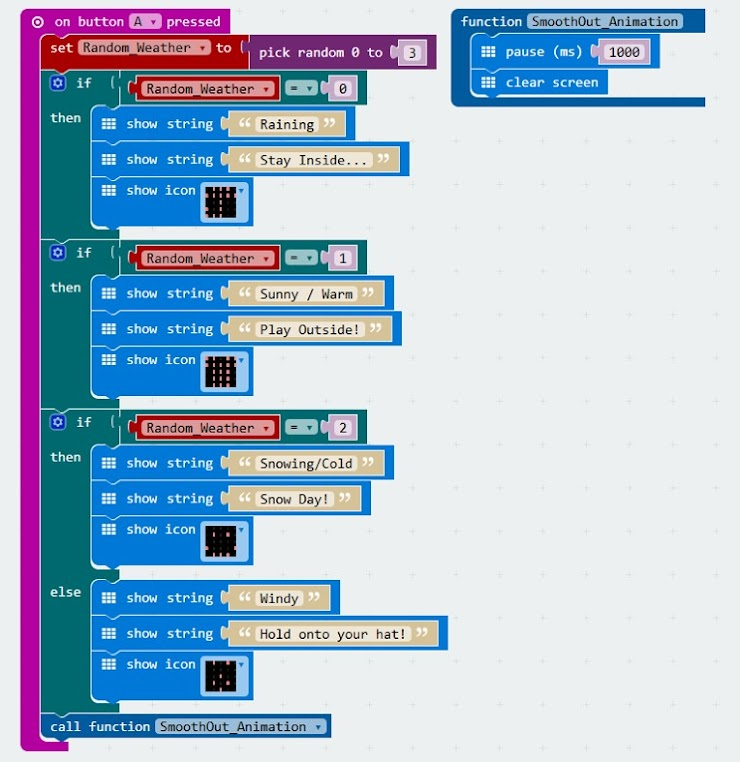Programming Selection (Microbit)
1. How many variables are used in the code below?
1 point2. What would be the first line of code displayed on the Microbit if the random number generated was 0?
1 point3. What would be the first line of code displayed on the Microbit if the random number generated was 1?
1 point4. What would be the first line of code displayed on the Microbit if the random number generated was 2?
1 point5. What would be the first line of code displayed on the Microbit if the random number generated was 3?
1 point6. What would be the first line of code to be displayed on the Microbit if the random number generated was 50?
1 point